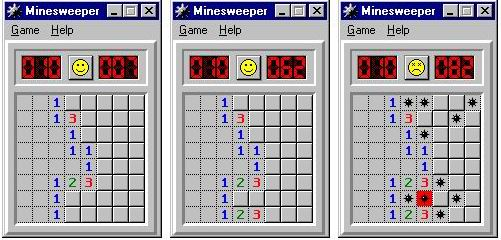poj2612,

## Mine Sweeper

 Time Limit: 1000MS Memory Limit: 65536K Total Submissions: 5122 Accepted: 2008
Description
The game Minesweeper is played on an n by n grid. In this grid are hidden mines, each at a distinct grid location. The player repeatedly touches grid positions. If a position with a mine is touched, the mine explodes and the player loses. If a positon not containing a mine is touched, an integer between 0 and 8 appears denoting the number of adjacent or diagonally adjacent grid positions that contain a mine. A sequence of moves in a partially played game is illustrated below.Here, n is 8, m is 10, blank squares represent the integer 0, raised squares represent unplayed positions, and the figures resembling asterisks represent mines. The leftmost image represents the partially played game. From the first image to the second, the player has played two moves, each time choosing a safe grid position. From the second image to the third, the player is not so lucky; he chooses a position with a mine and therefore loses. The player wins if he continues to make safe moves until only m unplayed positions remain; these must necessarily contain the mines. Your job is to read the information for a partially played game and to print the corresponding board.

Input
The first line of input contains a single postitive integer n <= 10. The next n lines represent the positions of the mines. Each line represents the contents of a row using n characters: a period indicates an unmined positon while an asterisk indicates a mined position. The next n lines are each n characters long: touched positions are denoted by an x, and untouched positions by a period. The sample input corresponds to the middle figure above.
Output
Your output should represent the board, with each position filled in appropriately. Positions that have been touched and do not contain a mine should contain an integer between 0 and 8. If a mine has been touched, all positions with a mine should contain an asterisk. All other positions should contain a period.
Sample Input
```8
...**..*
......*.
....*...
........
........
.....*..
...**.*.
.....*..
xxx.....
xxxx....
xxxx....
xxxxx...
xxxxx...
xxxxx...
xxx.....
xxxxx...```
` `
Sample Output
```001.....
0013....
0001....
00011...
00001...
00123...
001.....
00123...```
Source
Waterloo local 1999.10.02

## Source Code:

```
//Wed Mar  2 12:31:10 CST 2011
#include <vector>
#include <list>
#include <map>
#include <set>
#include <deque>
#include <queue>
#include <stack>
#include <bitset>
#include <algorithm>
#include <functional>
#include <numeric>
#include <utility>
#include <sstream>
#include <iostream>
#include <iomanip>
#include <cstdio>
#include <cmath>
#include <cstdlib>
#include <cctype>
#include <string>
#include <cstring>
#include <cstdio>
#include <cmath>
#include <cstdlib>
#include <ctime>

using namespace std;

char check(vector<string> v, int x, int y) {
int ret = 0;
int m = v.size();
int n = v.size();
if (v[x][y] == '*') return '*';
else {
if (x > 0 && v[x - 1][y] == '*') ret++;
if (x > 0 && y > 0 && v[x - 1][y - 1] == '*') ret++;
if (y > 0 && v[x][y - 1] == '*') ret++;
if (x + 1 < m && y > 0 && v[x + 1][y - 1] == '*') ret++;
if (x + 1 < m && v[x + 1][y] == '*') ret++;
if (x + 1 < m && y + 1 < n && v[x + 1][y + 1] == '*') ret++;
if (y + 1 < n && v[x][y + 1] == '*') ret++;
if (x > 0 && y + 1 < n && v[x - 1][y + 1] == '*') ret++;
}
return '0' + ret;
}

int main(int argc, const char* argv[]) {
//freopen("input.in", "r", stdin);
//freopen("output.out", "w", stdout);
int n;
while (cin >> n) {
bool flag = false;
vector<string> v1(n, string(n, '.'));
vector<string> v2(n, string(n, '.'));
for (int i = 0; i < n; i++)
cin >> v1[i];
for (int i = 0; i < n; i++) {
for (int j = 0; j < n; j++) {
char temp;
cin >> temp;
if (temp == 'x') {
if (v1[i][j] == '*') {
flag = true;
v2[i][j] = '*';
} else {
v2[i][j] = check(v1, i, j);
}
} else {
v2[i][j] = temp;
}
}
}
for (int i = 0; i < n; i++) {
for (int j = 0; j < n; j++) {
if (flag && v1[i][j] == '*')
cout << '*';
else
cout << v2[i][j];
}
cout << endl;
}
}
//fclose(stdin);
//fclose(stdout);
return 0;
}

```